Function Repository Resource:

# GaussianHessenbergDecomposition

Compute the Gaussian Hessenberg decomposition of a matrix

Contributed by: Jan Mangaldan
 ResourceFunction["GaussianHessenbergDecomposition"][m] gives the Gaussian Hessenberg decomposition of a square matrix m. ResourceFunction["GaussianHessenbergDecomposition"][{m,a}] gives the Gaussian Hessenberg-triangular decomposition of the matrix pencil {m,a}.

## Details

ResourceFunction["GaussianHessenbergDecomposition"][m] gives a result of the form {p,h}, where the matrices p and h satisfy the relation p.h.Inverse[p]==m.
In the Gaussian Hessenberg decomposition, the matrix h is an upper Hessenberg matrix that is similar to (in other words, has the same eigenvalues as) the original matrix, while the matrix p is a product of permutation matrices and stabilized elementary matrices (Gauss transforms).
ResourceFunction["GaussianHessenbergDecomposition"][{m,a}] yields a list of matrices {q,h,p,t}, where h is an upper Hessenberg matrix and t is an upper‐triangular matrix, such that m is given by q.h.p and a is given by q.t.p.
The matrices q and p in the Gaussian Hessenberg-triangular decomposition are products of permutation matrices and stabilized elementary matrices (Gauss transforms).

## Examples

### Basic Examples (2)

Find the Gaussian Hessenberg decomposition of a 4×4 matrix:

 In:=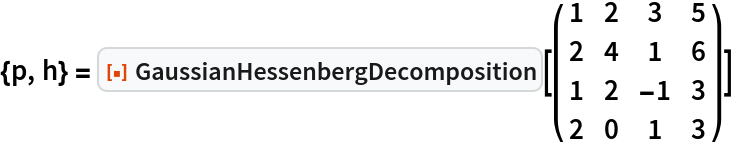Out=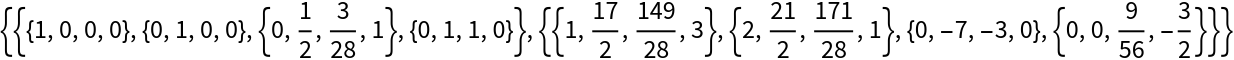The matrix h is an upper Hessenberg matrix:

 In:=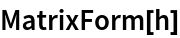Out=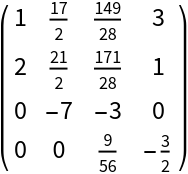### Scope (6)

The Gaussian Hessenberg decomposition for a complex matrix:

 In:=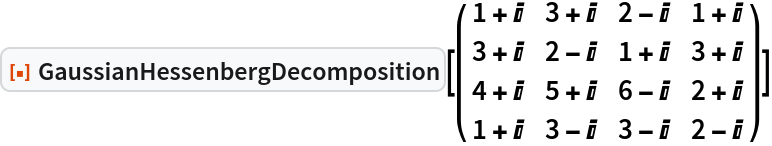Out=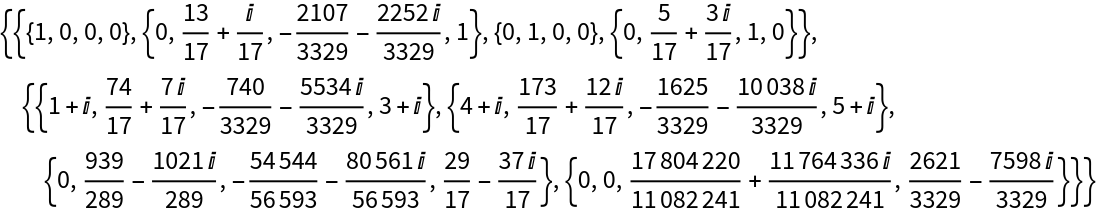In:=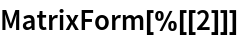Out=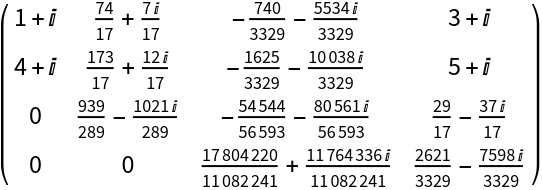The Gaussian Hessenberg decomposition for a numeric matrix:

 In:=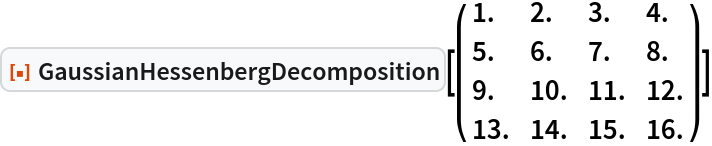Out=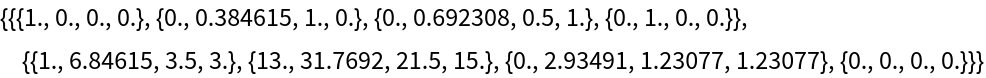In:=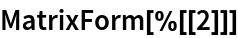Out=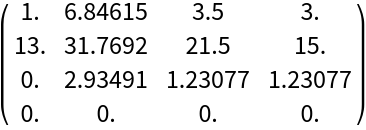The Gaussian Hessenberg decomposition for a matrix with entries having 24­digit precision:

 In:=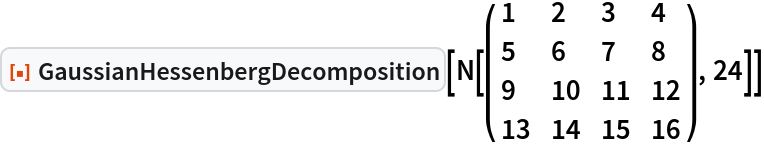Out=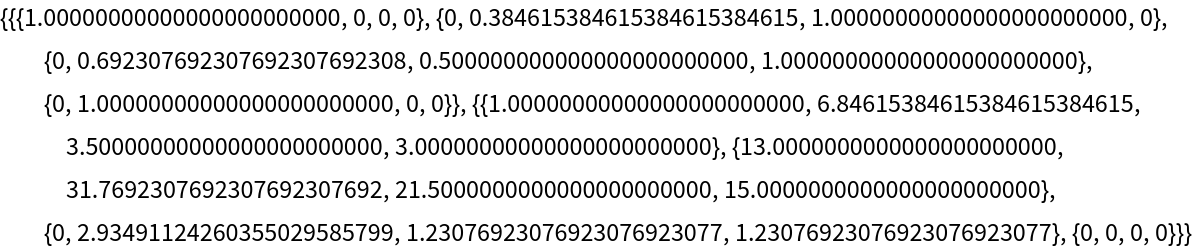The Gaussian Hessenberg decomposition for a symbolic matrix:

 In:=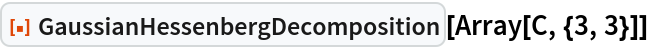Out=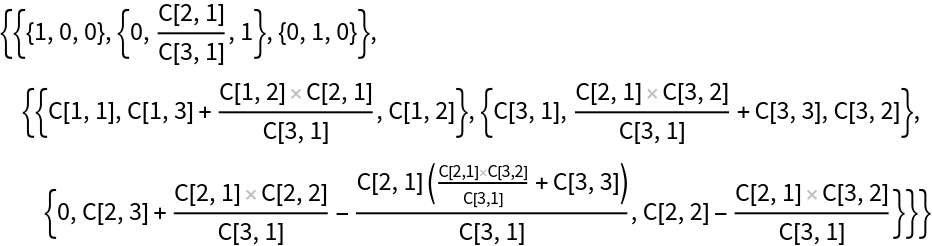The Gaussian Hessenberg-triangular decomposition for an exact matrix pencil:

 In:=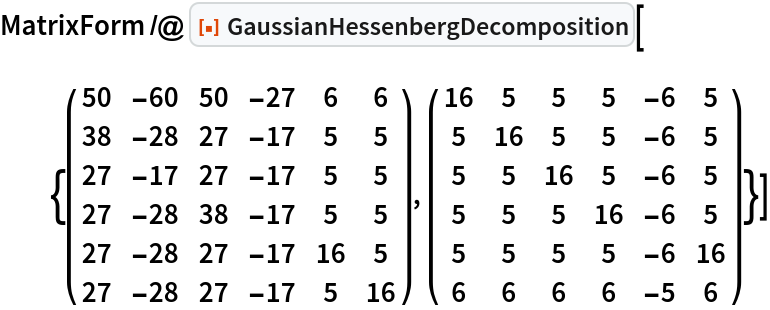Out=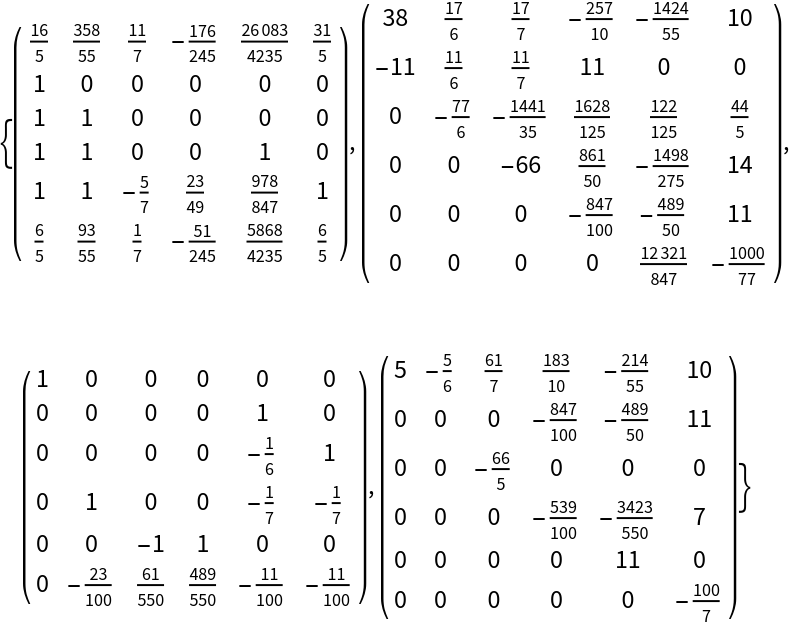The Gaussian Hessenberg-triangular decomposition for a numeric matrix pencil:

 In:=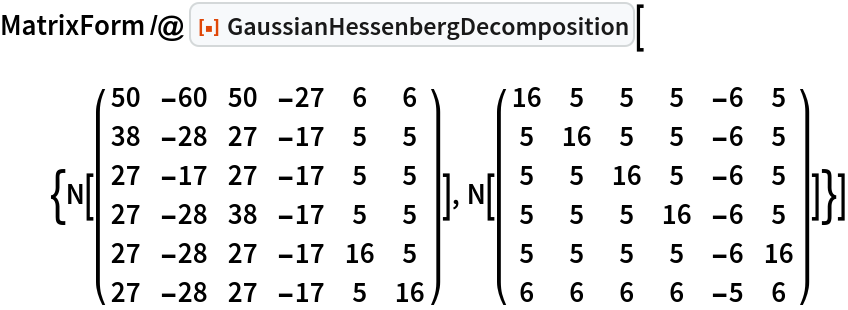Out=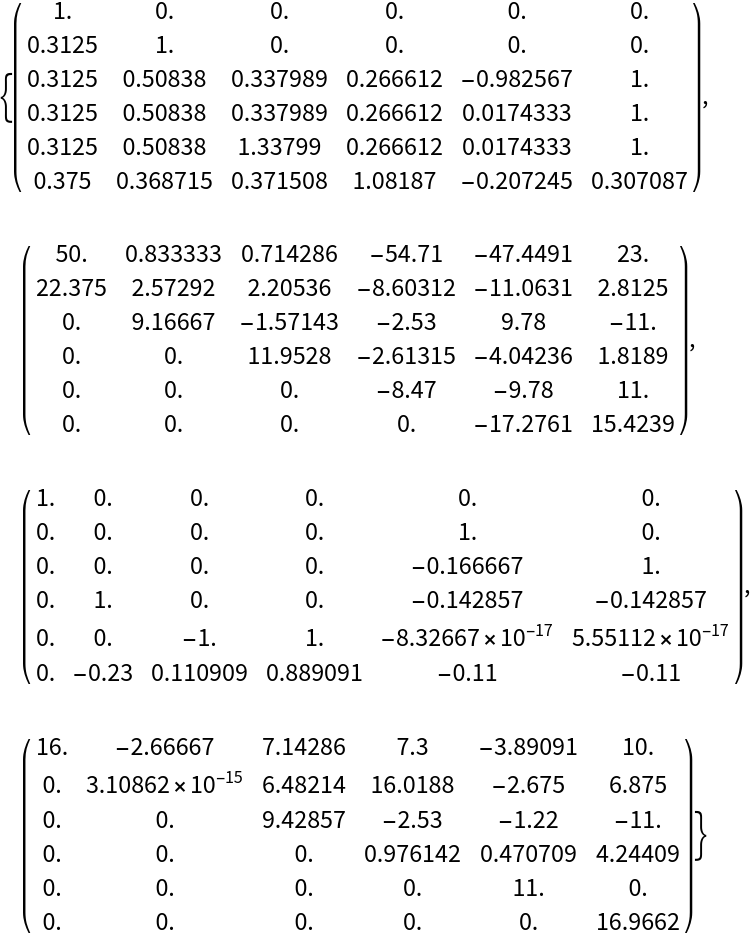### Properties and Relations (3)

A 4×4 matrix:

 In:=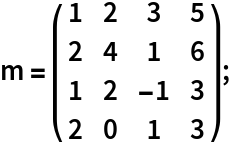Compute its Gaussian Hessenberg decomposition:

 In:=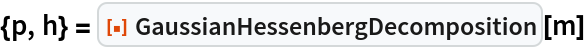Out=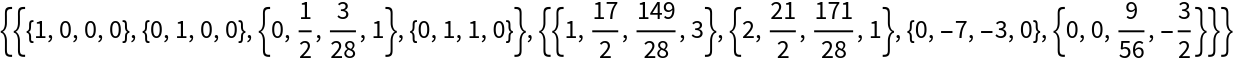The matrix h is upper Hessenberg:

 In:=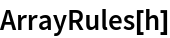Out=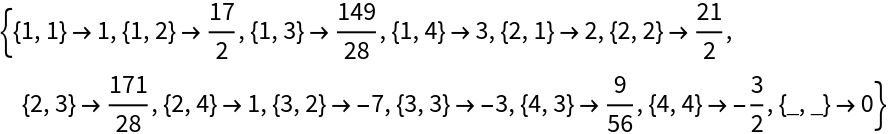In:=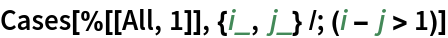Out=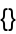The original matrix m is given by p.h.Inverse[p]:

 In:=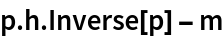Out=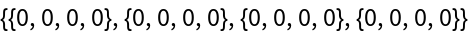A pair of 4×4 matrices:

 In:=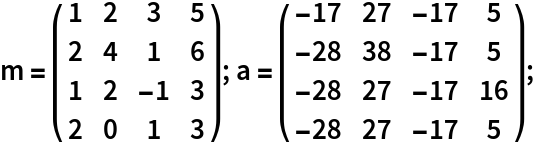Compute the Gaussian Hessenberg-triangular decomposition of the corresponding pencil:

 In:=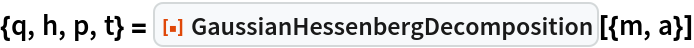Out=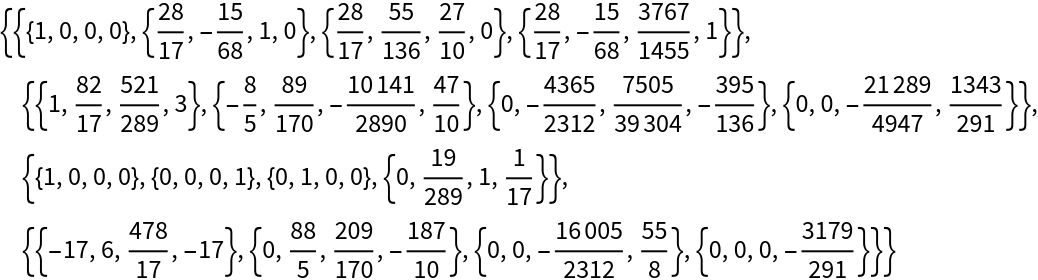The matrix h is upper Hessenberg:

 In:=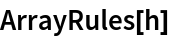Out=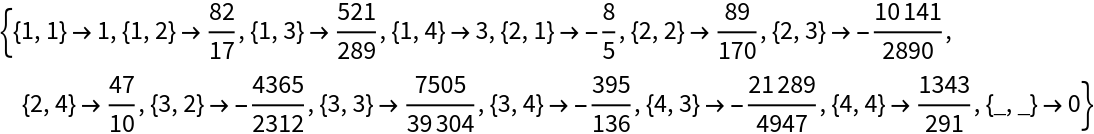In:=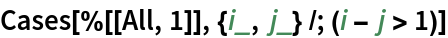Out=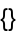The matrix t is upper triangular:

 In:=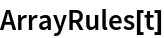Out=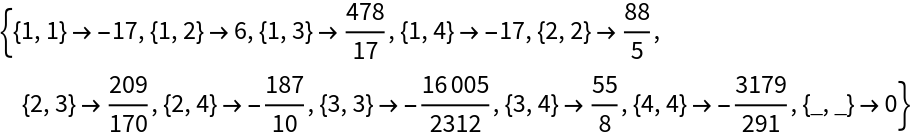In:=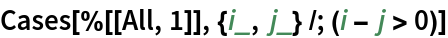Out=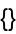The matrix m is given by q.h.p:

 In:=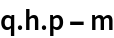Out=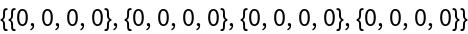The matrix a is given by q.t.p:

 In:=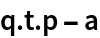Out=GaussianHessenbergDecomposition gives a different result from the built-in HessenbergDecomposition:

 In:=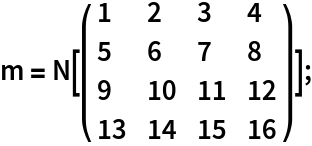In:=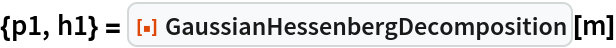Out=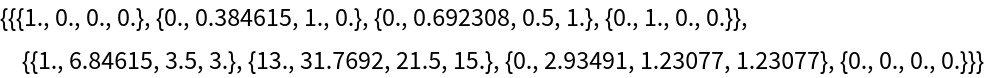In:=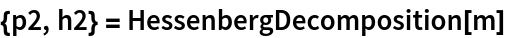Out=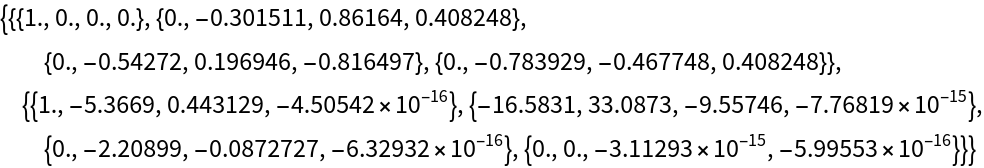Both decompositions yield upper Hessenberg matrices that are similar to the original matrix:

 In:=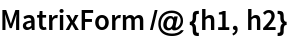Out=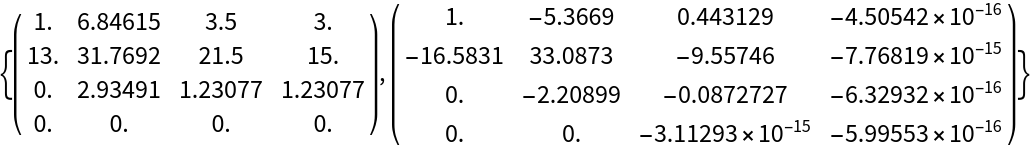HessenbergDecomposition yields a unitary transformation matrix, while GaussianHessenbergDecomposition does not:

 In:=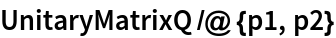Out=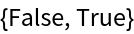## Version History

• 1.0.0 – 17 May 2021